# Getting started with Machine Learning and its Algorithms 2020.

Computer Skill with fire demand explained in an easy manner.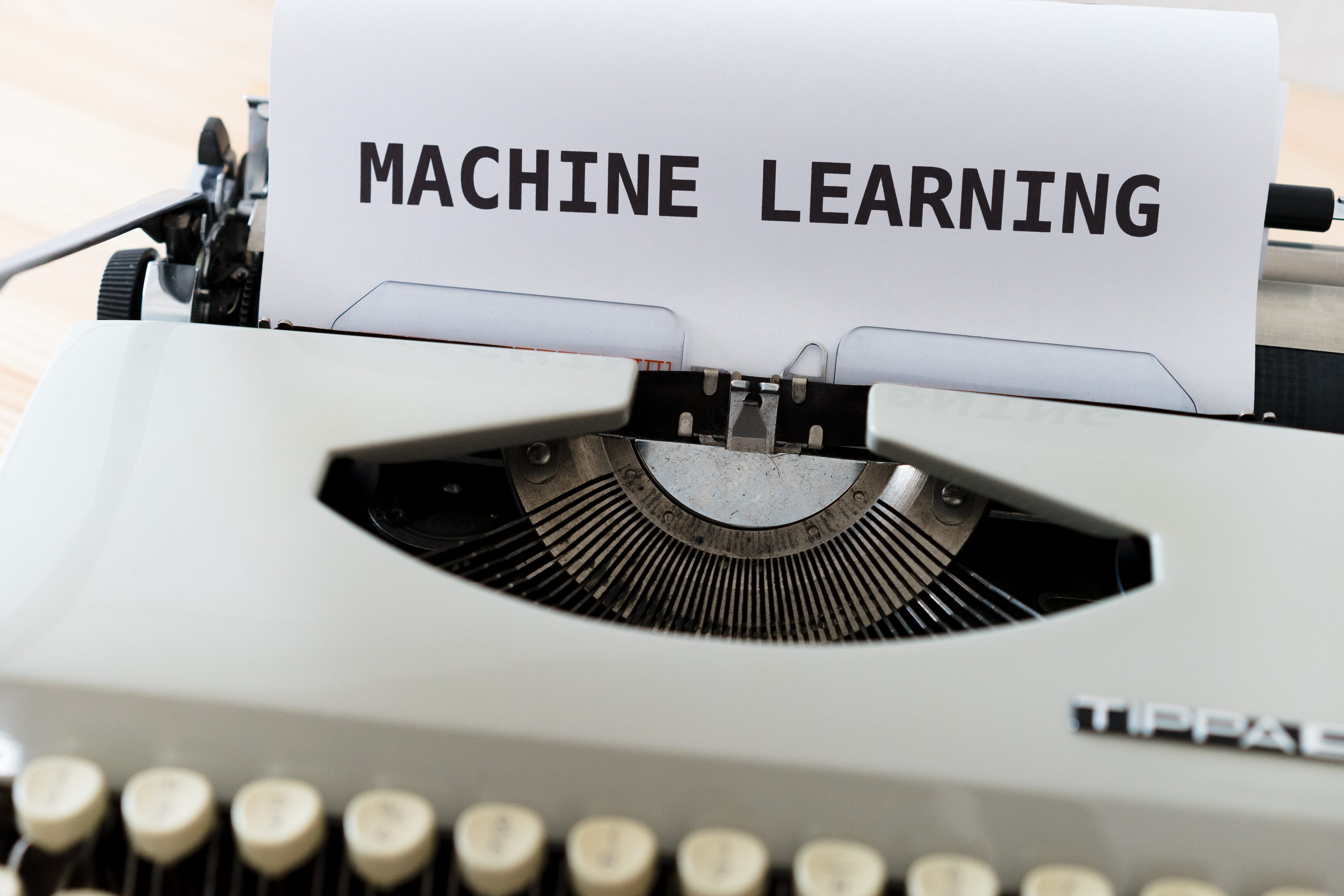Photo by Markus Winkler on Unsplash

ola Readers, From a future Engineer to other engineers out there,

Machine Learning a Buring topic, a much-needed skill to grab a suitable job in this present era of skill and development.

They say ML is hard, not easy, though it is but for a smart work and smart learner it isn’t. Developing new machine learning algorithms indeed involves a lot of maths and specialist knowledge. But most of the time doing machine learning is not about new algorithms, it is about using existing algorithms and code libraries to train machine learning models.

Now coming to What, Why, and others…

What is Machine Learning?

After hearing the term machine Learning…it sounds like a big Technical term but when you will get to know about it will seem like a simple concept to you which is usually getting used everywhere nowadays. It’s basically a type of learning in which machine starts learning things on its own without explicitly programmed. It is a subset of or a type of application of Artificial intelligence that provides the ability to the system to automatically learn from its past experiences and improve itself for coming processing.

Machine learning always works on the development of a computer program so it can access data and use that data for self-learning afterward. Machine learning aims at how computers learn automatically without any human intervention or any assistance so that the machine can adjust their actions according to need.

“A baby learns to crawl, walk and then run. We are in the crawling stage when it comes to applying machine learning.” ~Dave Waters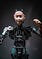Photo by Maximalfocus on Unsplash

# Prerequisite for machine learning :

Having prior knowledge of the following is necessary before learning machine learning.

1. Linear algebra

2. Calculus

3. Probability theory

4. Programming

5. Optimization theory

Though not that much necessary having a basic knowledge of all this will speed up your learning ability of ML.

# POPULAR ALGORITHMS IN MACHINE LEARNING

## 1. Linear Regression:

Linear regression is the most common and general algorithm in ML. Linear Regression(LR) algorithm, based on supervised learning; now what is supervised learning…Supervised learning is basically learning with the data, or in simple words, it means training or teaching the ML model using data/datasets which are labeled,i.e. defined datasets.

So, LR is basically training a model and after that, there comes testing the model with the sets of examples or test datasets so that our ML model analyses the training datasets and produces the correct output based on its supervised learning algorithms.
It performs Regression tasks…that means regression predictive modeling i.e to map or approximating a mapping function from the input variable say ‘x’ to a continuous variable say ‘y’.
It gives prediction value based on independent variables.
Its mainly used for finding out the relationship between variables and forecasting.
LR performs the task to predict the dependent variable ‘y’ based on the independent variable ‘x’. So it basically gives the linear relationship between ‘x’ and ‘y’ so-called linear variables.

Hypothesis function for LR: Y= θ1 +θ2.x

Here Y= labels of data(supervised learning)
x = input training data; parameter
θ1 = intercept
θ2 = coefficient of ‘x’
When train a model it fits the best line to predict the value of ‘y’ for a given value of ‘x’.
The model gets the best regression fit line with the best value of θ1 and θ2.

Cost functions(J) in LRcost functions are used to estimate how badly models are performing. Put simply, a cost function is a measure of how wrong the model is in terms of its ability to estimate the relationship between X and Y. This is typically expressed as a difference or distance between the predicted value and the actual value.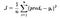The cost function in LR

In LR we predict such value of ‘ y’ such that error difference between the predicted value and true value is minimum.
Cost function(J) of Linear Regression is the Root Mean Squared Error (RMSE) between predicted y value (pred) and true y value (y).
It is used to minimize cost and maximize the productivity of an organization.

## 2. Decision Trees (DT):

The decision tree Machine learning algorithm is another supervised learning algorithm. They can be used to solve both Regression and Classification problems….in simple words, the task of the classification algorithm is to map the input variable ‘x’ for discrete output variable ‘y’.
DT is used both in Data mining and Ml…so we can say a famous algorithm.
The decision tree basically uses the tree representation to solve the problem in which each leaf node(Last nodes having no child) corresponds to a class labels say “cat” and attributes are represented on the internal node of the tree say “animal”.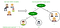https://www.geeksforgeeks.org/

One can't just ignore the simplicity of this algorithm…its often called Learning decision tree from data.
In general DT algorithm is referred to as a class and regression trees.
A classification tree is those whose generally targets is to classify possibilities or can better say yes/no outcomes. For Ex:- He eats or He not eats.
whereas Regression trees are those which predict continuous values. For Ex:- The price of the thing.
Growing a tree involves which features to choose and what conditions to use for splitting…along with the idea when to stop.
So, Recursive binary splitting used as a technique to split the tree…in this procedure, all the features are considered and different split points are tried and tested using a cost function.
we calculate how much accuracy a split cost us using a cost function, the lesser cost is chosen.
Cost Function in DT :
Try to find the most homogenous branch i.e having the same group with the same responses.

For Regression : sum(y-prediction)²

For classification : sum(pk* (1-pk))

In Decision Tree, the major challenge is to the identification of the attribute for the root node at each level. This process is known as attribute selection. We have two popular attribute selection measures:
Information Gain and the Gini Index measures.

## 3. K Nearest Neighbours(KNN):

K-Nearest Neighbors is also one of the most basic but essential classification algorithms in Machine Learning.
It is also based on a supervised learning algorithm that is discussed earlier.
It has its application in Data mining, pattern recognition,e.t.c.
It is greatly applicable in real-life scenarios.
Since it is non-parametric, meaning: it does not make any underlying assumptions about the distribution of data.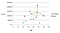https://www.saedsayad.com/k_nearest_neighbors.htm

In Figure, the arrows represent the nearest neighbors of the point.

The following two properties would define KNN well −

• Lazy learning algorithm − KNN is a lazy learning algorithm because it does not have a specialized training phase and uses all the data for training while classification.
• Non-parametric learning algorithm − KNN is also a non-parametric learning algorithm because it doesn’t assume anything about the underlying data.

We are given some prior data (also called training data) and also given another set of data points (also called testing data) and If we plot these points on a graph, we may be able to locate some clusters or groups. Now, given an unclassified point, we can assign it to a group by observing what group its nearest neighbors belong to. This means a point close to a cluster of points classified as ‘one class’ has a higher probability of getting classified as that very same ‘one class’.
The accuracy of this ML model increases as we increase the No of data points in the training dataset.

## 4.Random Forest (RF) :

It is also a supervised learning algorithm, flexible, and easy to use the algorithm. It has very little chance to go wrong also it doesn't go to overfitting problems(Overfitting happens when a model learns the detail and noise in the training data to the extent that it negatively impacts the performance of the model on new data).
It is slow in generating prediction as many DT is there and there is a possibility that a simple decision is time-consuming and complex but bias is prevented here as it takes an average of various voting.
It is used for both classification and regression techniques.
It comprises of tress, the more tree it has the robust the random forest is.
RF creates DT on randomly select data samples and gives prediction from each tress and selects the best solution on the basis of voting.
RF has a wide range of applications, some are image classification, recommendation engine, prediction of diseases, etc.

It basically works on 4 steps:-
— Select random samples from given datasets.
— Construct a DT for each sample and predict the result from each DT.
— Perform a vote for each predicted results.
— Select the prediction result with the most vote as the final prediction.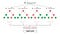https://blog.quantinsti.com/

It lies at the base of the Boruta algorithm….which means
it selects the important features in datasets
…wrap up build around Boruta algorithm, it tries to captures all the uninteresting features with respect to the outcome features.

## 5. Support Vector Machines (SVM) :

Support vector machines also termed as Support vector networks.
It is also a Supervised ML algorithm, used for both classification and regression challenges but used classification mostly nowadays.
An SVM model is a representation of the examples as points in space, mapped so that the examples of the separate categories are divided by a clear gap that is as wide as possible, formally defined by a separating hyperplane(the straight line that differentiates the two classes very well)…means we plot each data item as a point in n-dimensional space: n is the no of features, the value of each feature is the value of each coordinate. Then we perform the classification task by finding the hyperplane.

Now to decide the right hyperplane, we got some rules, they are:
— The hyperplane should be selected which segregate two classes better.
— Calculate the margin which is the distance between the nearest data point and hyper-plane. The plane has the maximum distance will be considered as the right hyperplane to classify the classes better.
SVM has rule 1 more dominant.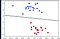Here the straight line is Hyperline which separates the two classes.

When we get an out-layer(a point/class at a different position from its members i.e. lying far away from its own kind),
then SVM takes maximum no of data points and maximizes them.
We can even go to non-linear hyperplane by adding a new feature or a new axis, and for this, the SVM has the kernel trick a function that takes a takes low dimensional input space into high dimensional output space.

Some most commonly used kernels are as follows:
1. Linear kernel for straight hyperplane.
2. Polynomial kernel for curved and non-linear hyperplane.
3. Radial basis function kernel…commonly used in the SVM machine.Photo by h heyerlein on Unsplash

“Technical content writer”

## More from Amandeep

“Technical content writer”